Electron. J. Diff. Eqns., Vol. 2004(2004), No. 59, pp. 1-30.

### Damped second order linear differential equation with deviating arguments: Sharp results in oscillation properties Leonid Berezansky & Yury Domshlak

Abstract:
This article presents a new approach for investigating the oscillation properties of second order linear differential equations with a damped term containing a deviating argument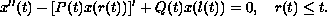To study this equation, a specially adapted version of Sturmian Comparison Method is developed and the following results are obtained:
(a) A comprehensive description of all critical (threshold) states with respect to its oscillation properties for a linear autonomous delay differential equation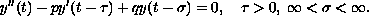(b) Two versions of Sturm-Like Comparison Theorems. Based on these Theorems, sharp conditions under which all solutions are oscillatory for specific realizations of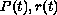and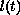are obtained. These conditions are formulated as the unimprovable analogues of the classical Knezer Theorem which is well-known for ordinary differential equations (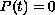,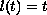).
(c) Upper bounds for intervals, where any solution has at least one zero.

Submitted March 23, 2004. Published April 19, 2004.
Math Subject Classifications: 34K11
Key Words: Linear differential equation with deviating arguments, second order, damping term, oscillation, Sturmian comparison method

Show me the PDF file (373K), TEX file, and other files for this article.Leonid Berezansky Department of Mathematics Ben-Gurion University of the Negev Beer-Sheva 84105, Israel e-mail: brznsky@cs.bgu.ac.il Yury Domshlak Department of Mathematics Ben-Gurion University of the Negev Beer-Sheva 84105, Israel e-mail: domshlak@cs.bgu.ac.il